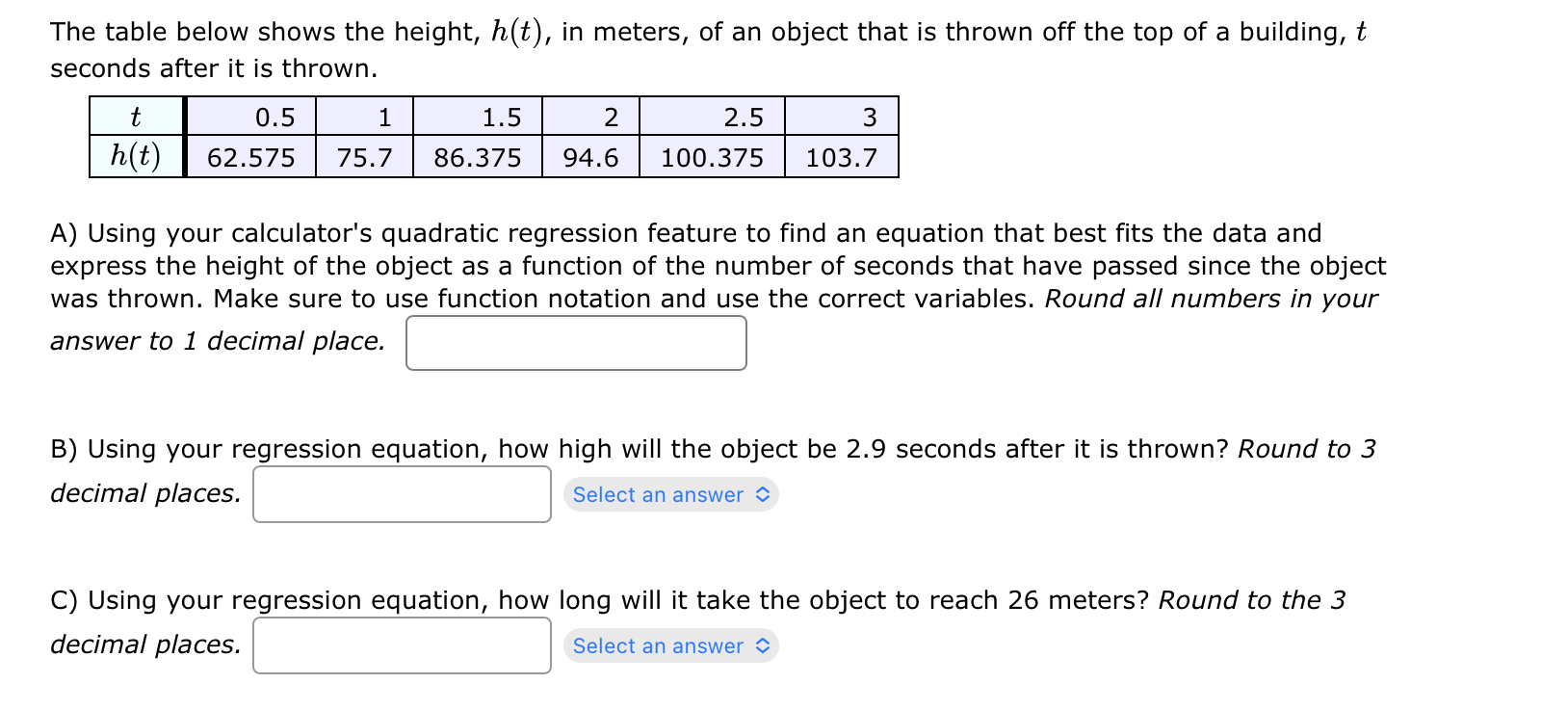Home / Expert Answers / Algebra / the-table-below-shows-the-height-h-t-in-meters-of-an-object-that-is-thrown-off-the-top-of-a-bui-pa299

# (Solved): The table below shows the height, h(t), in meters, of an object that is thrown off the top of a bui ...The table below shows the height, , in meters, of an object that is thrown off the top of a building, seconds after it is thrown. A) Using your calculator's quadratic regression feature to find an equation that best fits the data and express the height of the object as a function of the number of seconds that have passed since the object was thrown. Make sure to use function notation and use the correct variables. Round all numbers in your answer to 1 decimal place. B) Using your regression equation, how high will the object be 2.9 seconds after it is thrown? Round to 3 decimal places. C) Using your regression equation, how long will it take the object to reach 26 meters? Round to the 3 decimal places.

We have an Answer from Expert